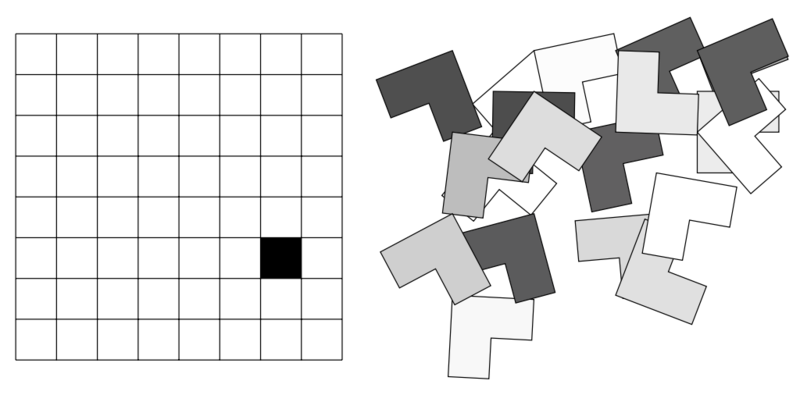over 3 years ago

## 2 直接找出第 $i$ 步該做什麼

Step A B C 備註
0 (000) $\{1,2,3\}$ $\{\}$ $\{\}$ 初始狀態
1 (001) $\{2,3\}$ $\{\}$ $\{1\}$ $(1, A\to C)$
2 (010) $\{3\}$ $\{2\}$ $\{1\}$ $(2, A\to B)$
3 (011) $\{3\}$ $\{1,2\}$ $\{\}$ $(1, C\to B)$
4 (100) $\{\}$ $\{1,2\}$ $\{3\}$ $(3, A\to C)$
5 (101) $\{1\}$ $\{2\}$ $\{3\}$ $(1, B\to A)$
6 (110) $\{1\}$ $\{\}$ $\{2,3\}$ $(2, B\to C)$
7 (111) $\{\}$ $\{\}$ $\{1,2,3\}$ $(1, A\to C)$

over 3 years ago

## 2 紙條與搜索範圍

### 延伸練習

PA2011 Round 5 - Trails：這題比前一題更複雜一點點，但是方法應該是類似的。歡迎大家一起討論怎麼實作會更理想與簡潔！

over 3 years ago

## 2 解法

### 思考問題

1. 如果史萊姆的大小是 $2^k\times 2^k$，在什麼樣的條件下我們可以保證 L形磚一定可以鋪滿盤面的其他地方？
2. 證明存在一個 $16\times 16$ 缺一角的 L形磚鋪法，至少要用四種顏色塗每一片磚，使得有邊相鄰的兩塊磚顏色都不相同。（即，這個鋪磚法無法三著色。）說不定有 $8\times 8$ 的構造法？（三著布拉克）

## 5 奇怪的後記over 3 years ago

## 4 參考資料

http://theory.stanford.edu/~virgi/cs367/lecture3-56.pdf

over 3 years ago

## 1 維度更高的時候怎麼辦？

### 複雜度分析

$d=1$$d=2$ 的時候，我們可以用 $O(n\log n)$ 的時間解決這題。假若我們要解決的問題是 $n$ 個點、$d$ 維的問題。可以得到遞迴式：

over 3 years ago

## 3 STL

http://en.cppreference.com/w/cpp/algorithm/nth_element

over 3 years ago

over 3 years ago

## 0 緣起

Divide and Conquer：分而治之法，又稱為各個擊破法。基本上就是把一個問題分成很多子問題，然後各自解決這些子題目（通常是用遞迴方法），接著再把這些子題目組合得出原本問題的解。

## 1 快速冪問題

### 說明

$n=0$ 的時候，我們知道答案會是 $1$（若 $x\neq 0$）。當 $n > 0$ 的時候，我們知道如果 $n$ 是偶數，那麼只要能夠算出 $a = x^{n/2}$，就可以得出 $a^2=x^n$。若 $n$ 是奇數，則我們可以先算出 $a=x^{(n-1)/2}$，然後利用 $a^2x=x^n$ 就可以得到我們要的解了。

## 4 思考問題

1. 如果我們今天不模 $p$ 取餘數，要做大數計算，請問「先遞迴再平方」跟「先平方再遞迴」何者效率較佳？為什麼？
2. 如果今天有一個比較快的多項式相乘作法（請參考 CLRS 第 30 章），$f(d_1, d_2) = (d_1+d_2)\log (d_1+d_2)$，那麼「先遞迴再平方」與「先平方再遞迴」的時間複雜度分別為何？

over 5 years ago

## Hello World

Hi, This a demo post of Logdown.

Logdown use Markdown as main syntax, you can find more example by reading this document on Wikipedia

Logdown also support drag & drop image uploading. The picture syntax is like this:

inline code

### MathJax Example

#### Inline Mathjax

The answser is $a^2 + b^2 = c^2$.

### Table Example

Tables Are Cool
col 1 Hello $1600 col 2 Hello$12
col 3 Hello \$1